1596458460

# Ridge Regression: Regularization Fundamentals

Regularization is a method used to reduce the variance of a Machine Learning model; in other words, it is used to reduce overfitting. Overfitting occurs when a machine learning model performs well on the training examples but fails to yield accurate predictions for data that it has not been trained on.

In theory, there are 2 major ways to build a machine learning model with the ability to generalize well on unseen data:

1. Train the simplest model possible for our purpose(according to Occam’s Razor).
2. Train a complex or more expressive model on the data and perform regularization.

It has been observed that method #2 yields the best performing models by contemporary standards. In other words, we want our model to have the ability to capture highly complex functions. However, to overcome overfitting, we regularize it.

# Objective:

In the present article we will discuss:

1. Effect of regularization on coefficients and model performance.
2. Data pre-processing steps mandatory for regularization.

We will use the Boston Housing Prices Data available in scikit-learn.

# Data

``````import pandas as pd
import numpy as np
import matplotlib.pyplot as plt
from sklearn import preprocessing, linear_model, model_selection, metrics, datasets, base
# Load LSTAT and RM Features from Boston Housing Data
X = pd.DataFrame(bos.data, columns = bos.feature_names)[['LSTAT', 'RM']]
y = bos.target
``````

# Effect of regularization on Model Coefficients

Regularization penalizes a model for being more complex; for linear models, it means regularization forces model coefficients to be smaller in magnitude.

First let us understand the problems of having large model coefficients. Let us assume a linear model trained on the above data. Let us assume the regression coefficient for the input LSTAT to be large. Now, this means, that assuming all the features are scaled, for a very small change in LSTAT, the prediction will change by a large amount. This simply follows from the Equation for Linear Regression.

In general, inputs having significantly large coefficients tend to drive the model predictions when all the features take values in similar ranges. This becomes a problem if the important feature is noisy or the model overfits to the data — because this causes the model predictions to be either driven by noise or by insignificant variations in LSTAT.

In other words, in general, we want the model to to have coefficients of smaller magnitudes.

Let us See if regularizing indeed reduces the magnitude of coefficients. To visualize this, we will generate polynomial features from our data of all orders from 1 to 10 and make a box-plot of the magnitude of coefficients of the features for:

1. Un-regularized Linear Regression
2. L2 Regularized Linear Regression(Ridge)

Note: Before fitting the model, we are standardizing the inputs.

``````model = linear_model.LinearRegression()
scaler = preprocessing.StandardScaler().fit(X_train)
X_scaled = scaler.transform(X_train)
model.fit(X_scaled , y_train)
coefs = pd.DataFrame()
coefs['Features'] = X.columns
coefs['1'] = np.abs(model.coef_)
for order in range(2, 11):
poly = preprocessing.PolynomialFeatures(order).fit(X_train)
X_poly = poly.transform(X_train)
scaler = preprocessing.StandardScaler().fit(X_poly)
model = linear_model.LinearRegression().fit(scaler.transform(X_poly), y_train)
coefs = pd.concat([coefs, pd.Series(np.abs(model.coef_), name = str(order))], axis = 1)

sns.boxplot(data = pd.melt(coefs.drop('Features', axis = 1)), x = 'variable', y = 'value',
order = [str(i) for i in range(1, 11)], palette = 'Blues')
ax = plt.gca()
ax.yaxis.grid(True, alpha = .3, color = 'grey')
ax.xaxis.grid(False)
plt.yscale('log')
plt.xlabel('Order of Polynomial', weight = 'bold')
plt.ylabel('Magnitude of Coefficients', weight = 'bold')
``````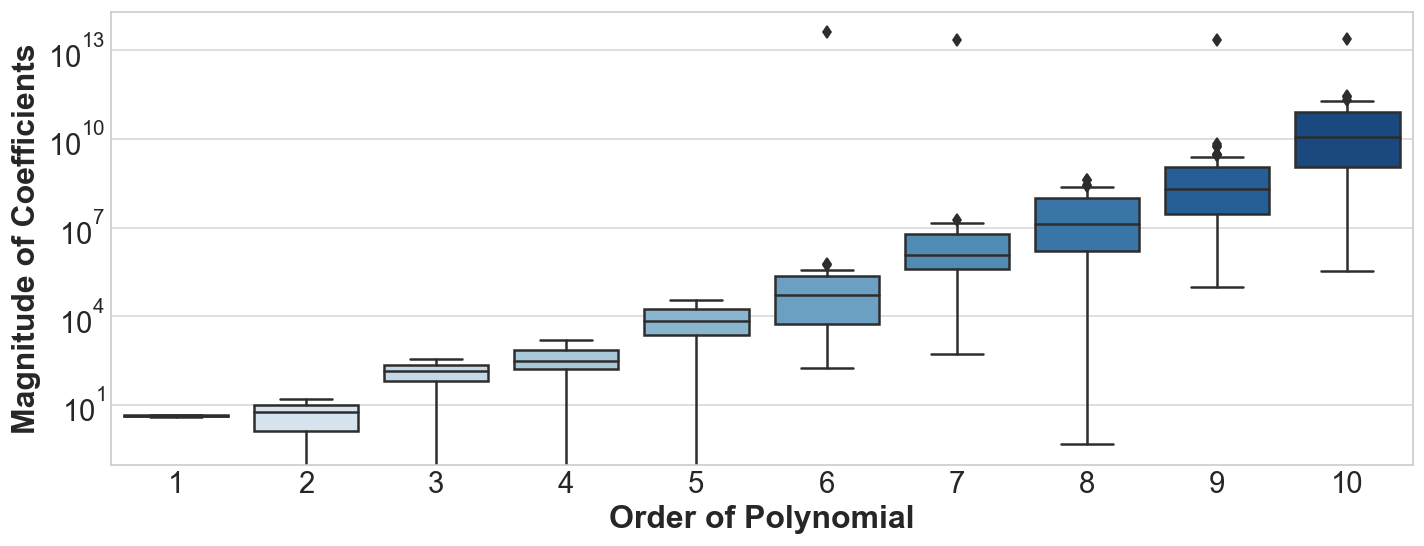Distribution of Linear Model(Not Regularized) Coefficients for polynomials of various degrees

We observe the following:

1. As the order of polynomial increases, the linear model coefficients become more likely to take on large values.
2. The largest coefficient of the 10th order polynomial is over 10¹² times the magnitude of the largest coefficient of the first order features.
3. Most of the higher order polynomials have coefficients in the order of 10⁴ to 10¹⁰

Let us now, perform the same exercise with Ridge(L2 Regularized) Regression.

#regression #linear-regression #scikit-learn #regularization #ridge-regression #deep learning

## Buddha Community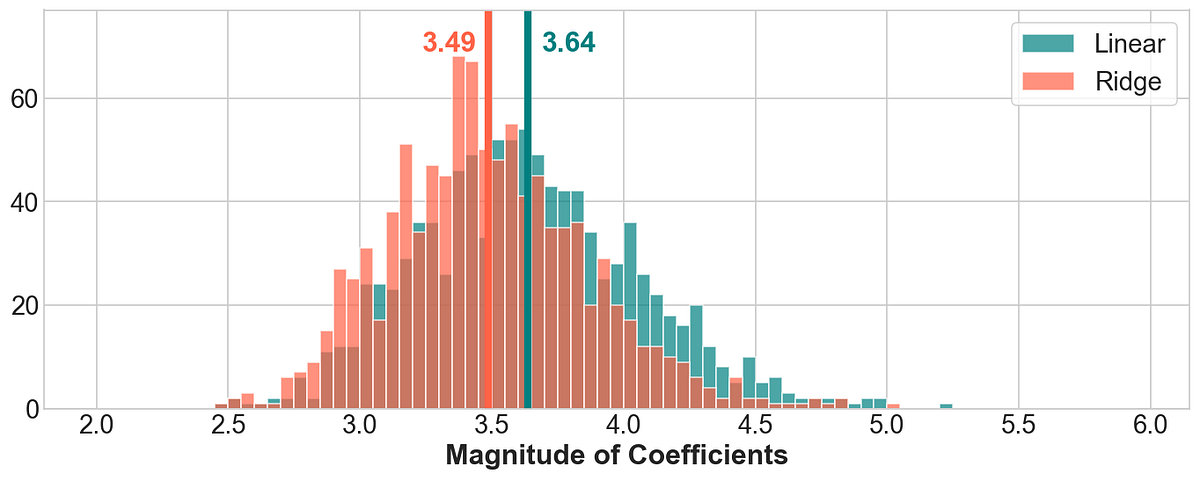1596458460

## Ridge Regression: Regularization Fundamentals

Regularization is a method used to reduce the variance of a Machine Learning model; in other words, it is used to reduce overfitting. Overfitting occurs when a machine learning model performs well on the training examples but fails to yield accurate predictions for data that it has not been trained on.

In theory, there are 2 major ways to build a machine learning model with the ability to generalize well on unseen data:

1. Train the simplest model possible for our purpose(according to Occam’s Razor).
2. Train a complex or more expressive model on the data and perform regularization.

It has been observed that method #2 yields the best performing models by contemporary standards. In other words, we want our model to have the ability to capture highly complex functions. However, to overcome overfitting, we regularize it.

# Objective:

In the present article we will discuss:

1. Effect of regularization on coefficients and model performance.
2. Data pre-processing steps mandatory for regularization.

We will use the Boston Housing Prices Data available in scikit-learn.

# Data

``````import pandas as pd
import numpy as np
import matplotlib.pyplot as plt
from sklearn import preprocessing, linear_model, model_selection, metrics, datasets, base
# Load LSTAT and RM Features from Boston Housing Data
X = pd.DataFrame(bos.data, columns = bos.feature_names)[['LSTAT', 'RM']]
y = bos.target
``````

# Effect of regularization on Model Coefficients

Regularization penalizes a model for being more complex; for linear models, it means regularization forces model coefficients to be smaller in magnitude.

First let us understand the problems of having large model coefficients. Let us assume a linear model trained on the above data. Let us assume the regression coefficient for the input LSTAT to be large. Now, this means, that assuming all the features are scaled, for a very small change in LSTAT, the prediction will change by a large amount. This simply follows from the Equation for Linear Regression.

In general, inputs having significantly large coefficients tend to drive the model predictions when all the features take values in similar ranges. This becomes a problem if the important feature is noisy or the model overfits to the data — because this causes the model predictions to be either driven by noise or by insignificant variations in LSTAT.

In other words, in general, we want the model to to have coefficients of smaller magnitudes.

Let us See if regularizing indeed reduces the magnitude of coefficients. To visualize this, we will generate polynomial features from our data of all orders from 1 to 10 and make a box-plot of the magnitude of coefficients of the features for:

1. Un-regularized Linear Regression
2. L2 Regularized Linear Regression(Ridge)

Note: Before fitting the model, we are standardizing the inputs.

``````model = linear_model.LinearRegression()
scaler = preprocessing.StandardScaler().fit(X_train)
X_scaled = scaler.transform(X_train)
model.fit(X_scaled , y_train)
coefs = pd.DataFrame()
coefs['Features'] = X.columns
coefs['1'] = np.abs(model.coef_)
for order in range(2, 11):
poly = preprocessing.PolynomialFeatures(order).fit(X_train)
X_poly = poly.transform(X_train)
scaler = preprocessing.StandardScaler().fit(X_poly)
model = linear_model.LinearRegression().fit(scaler.transform(X_poly), y_train)
coefs = pd.concat([coefs, pd.Series(np.abs(model.coef_), name = str(order))], axis = 1)

sns.boxplot(data = pd.melt(coefs.drop('Features', axis = 1)), x = 'variable', y = 'value',
order = [str(i) for i in range(1, 11)], palette = 'Blues')
ax = plt.gca()
ax.yaxis.grid(True, alpha = .3, color = 'grey')
ax.xaxis.grid(False)
plt.yscale('log')
plt.xlabel('Order of Polynomial', weight = 'bold')
plt.ylabel('Magnitude of Coefficients', weight = 'bold')
``````Distribution of Linear Model(Not Regularized) Coefficients for polynomials of various degrees

We observe the following:

1. As the order of polynomial increases, the linear model coefficients become more likely to take on large values.
2. The largest coefficient of the 10th order polynomial is over 10¹² times the magnitude of the largest coefficient of the first order features.
3. Most of the higher order polynomials have coefficients in the order of 10⁴ to 10¹⁰

Let us now, perform the same exercise with Ridge(L2 Regularized) Regression.

#regression #linear-regression #scikit-learn #regularization #ridge-regression #deep learning1593294180

## Regularization with Ridge, Lasso, and Elastic Net Regressions

Overview of the differences in 3 common regularization techniques — Ridge, Lasso, and Elastic Net.

#regression #regularization #ridge #lasso #elastic net regressions #.net1601172000

## L1 vs L2 Regularization and when to use which?

I have read many articles on the topic to find out which is better out of two and what should I use for my model. I wasn’t satisfied with any of them and that left my brain confused which one should I use? After having done so many experiments, I have finally found out all answers to Which Regularization technique to use and when? Let’s get to it using a regression example.

Let’s suppose we have a regression model for predicting y-axis values based on the x-axis value.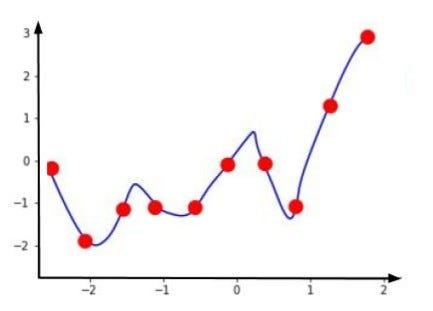Train Data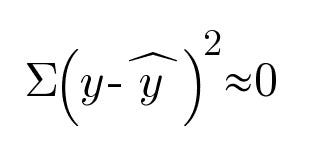Cost Function

While training the model, we always try to find the cost function. Here, y is the actual output variable and K is the predicted output. So, for the training data, our cost function will almost be zero as our prediction line passes perfectly from the data points.

Now, suppose our test dataset looks like as follows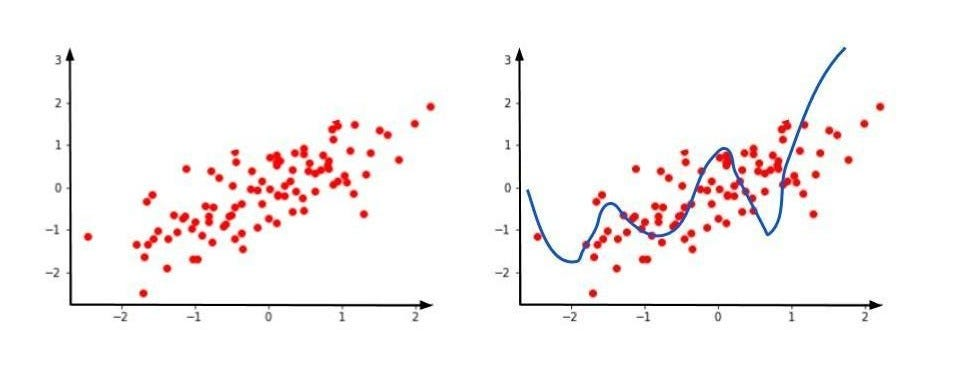Model on the test dataset

Here, clearly our prediction is somewhere else and the prediction line is directed elsewhere. This leads to overfitting. Overfitting says that with respect to training dataset you are getting a low error, but with respect to test dataset, you are getting high error.

Remember, when we need to create any model let it be regression, classification etc. It should be generalized.

We can use L1 and L2 regularization to make this overfit condition which is basically high variance to low variance. A generalized model should always have low bias and low variance.

#ridge-regression #data-science #machine-learning #regularization #lasso-regression1593001493

## Regularization with Ridge, Lasso, and Elastic Net Regressions

Overview of the differences in 3 common regularization techniques — Ridge, Lasso, and Elastic Net.

#regularization #regression #machine-learning #data-science #lasso #programming1598352300

## Regression: Linear Regression

Machine learning algorithms are not your regular algorithms that we may be used to because they are often described by a combination of some complex statistics and mathematics. Since it is very important to understand the background of any algorithm you want to implement, this could pose a challenge to people with a non-mathematical background as the maths can sap your motivation by slowing you down.In this article, we would be discussing linear and logistic regression and some regression techniques assuming we all have heard or even learnt about the Linear model in Mathematics class at high school. Hopefully, at the end of the article, the concept would be clearer.

**Regression Analysis **is a statistical process for estimating the relationships between the dependent variables (say Y) and one or more independent variables or predictors (X). It explains the changes in the dependent variables with respect to changes in select predictors. Some major uses for regression analysis are in determining the strength of predictors, forecasting an effect, and trend forecasting. It finds the significant relationship between variables and the impact of predictors on dependent variables. In regression, we fit a curve/line (regression/best fit line) to the data points, such that the differences between the distances of data points from the curve/line are minimized.

#regression #machine-learning #beginner #logistic-regression #linear-regression #deep learning